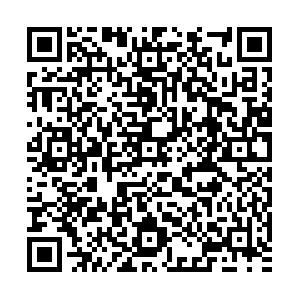# Ratio of a strange quark mass ms to up or down quark mass mu,d predicted by a quark propagator in the frameworkof the chiral perturbation theory

• Based on the fully dressed quark propagator and chiral perturbation theory, we study the ratio of the strange quark mass ms to up or down quark mass mu,d. The ratio is related to the determination of quark masses which are fundamental input parameters of QCD Lagrangian in the Standard Model of particle physics and can not be directly measured since the quark is confined within a hadron. An accurate determination of these QCD free parameters is extremely important for both phenomenological and theoretical applications. We begin with a brief introduction to the non-perturbation QCD theory, and then study the mass ratio in the framework of the chiral perturbation theory (χ PT) with a parameterized fully dressed quark propagator which describes confining fully dressed quark propagation and is analytic everywhere in the finite complex p2-plane and has no Lehmann representation so there are no quark production thresholds in any theoretical calculations of observable data. Our prediction for the ratio ms/mu,d is consistent with other model predictions such as Lattice QCD, instanton model, QCD sum rules and the empirical values used widely in the literature. As a by-product of this study, our theoretical results, together with other predictions of physical quantities that used this quark propagator in our previous publications, clearly show that the parameterized form of the fully dressed quark propagator is an applicable and reliable approximation to the solution of the Dyson-Schwinger Equation of quark propagator in the QCD.
PCAS:
••Get Citation
PENG Jin-Song, ZHOU Li-Juan, MENG Cheng-Ju, PAN Ji-Huan, MA Wei-Xing and YUAN Tong-Quan. Ratio of a strange quark mass ms to up or down quark mass mu,d predicted by a quark propagator in the frameworkof the chiral perturbation theory[J]. Chinese Physics C, 2013, 37(4): 043101. doi: 10.1088/1674-1137/37/4/043101
PENG Jin-Song, ZHOU Li-Juan, MENG Cheng-Ju, PAN Ji-Huan, MA Wei-Xing and YUAN Tong-Quan. Ratio of a strange quark mass ms to up or down quark mass mu,d predicted by a quark propagator in the frameworkof the chiral perturbation theory[J]. Chinese Physics C, 2013, 37(4): 043101.Milestone
Revised: 2012-11-20
Article Metric

Article Views(1467)
Cited by(0)
Policy on re-use
To reuse of subscription content published by CPC, the users need to request permission from CPC, unless the content was published under an Open Access license which automatically permits that type of reuse.
###### 通讯作者: 陈斌, bchen63@163.com
• 1.

沈阳化工大学材料科学与工程学院 沈阳 110142

Title:
Email:

## Ratio of a strange quark mass ms to up or down quark mass mu,d predicted by a quark propagator in the frameworkof the chiral perturbation theory

Abstract: Based on the fully dressed quark propagator and chiral perturbation theory, we study the ratio of the strange quark mass ms to up or down quark mass mu,d. The ratio is related to the determination of quark masses which are fundamental input parameters of QCD Lagrangian in the Standard Model of particle physics and can not be directly measured since the quark is confined within a hadron. An accurate determination of these QCD free parameters is extremely important for both phenomenological and theoretical applications. We begin with a brief introduction to the non-perturbation QCD theory, and then study the mass ratio in the framework of the chiral perturbation theory (χ PT) with a parameterized fully dressed quark propagator which describes confining fully dressed quark propagation and is analytic everywhere in the finite complex p2-plane and has no Lehmann representation so there are no quark production thresholds in any theoretical calculations of observable data. Our prediction for the ratio ms/mu,d is consistent with other model predictions such as Lattice QCD, instanton model, QCD sum rules and the empirical values used widely in the literature. As a by-product of this study, our theoretical results, together with other predictions of physical quantities that used this quark propagator in our previous publications, clearly show that the parameterized form of the fully dressed quark propagator is an applicable and reliable approximation to the solution of the Dyson-Schwinger Equation of quark propagator in the QCD.

### HTML

Reference (1)
PDF查看关注分享

Top

### 目录/DownLoad:  Full-Size Img  PowerPoint